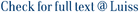Computing the LZ77 factorization is a fundamental task in text compression and indexing, being the size z of this compressed representation closely related to the self-repetitiveness of the text. A long-standing problem is to compute LZ77 using small working space. Considering that O(z) words of space can be significantly (up to exponentially) smaller than the size n of the input text, even succinct and entropycompressed solutions are often unduly memory demanding. In this work we focus on an important measure of text repetitiveness: the number r of equal-letter runs in the Burrows–Wheeler transform of the reversed input text. As z, the measure r is closely related to the number of repetitions in the text and can be exponentially smaller than n. We describe two algorithms computing LZ77 in O(r log n) bits of working space and O(n log r) time. Roughly speaking, our algorithms store a constant number of memory words per BWT run to keep track of first-last run-positions and a suitable indexing mechanism to sample the runs of the BWT (instead of its positions). Important consequences of our results include (i) the possibility to convert from RLBWT- to LZ77-based compressed formats without first decompressing the text, and (ii) the existence of asymptotically-optimal construction algorithms for repetition-aware selfindexes based on these compression techniques.We finally describe an implementation of our solutions and present extensive experiments on highly repetitive datasets. Our algorithms use a working space as small as 1% of the dataset size and are two to three orders of magnitude more space-efficient (albeit slower) than existing solutions based, respectively, on entropy compression and suffix arrays.

LZ77 Computation Based on the Run-Length Encoded BWT / Policriti, Alberto; Prezza, Nicola. - In: ALGORITHMICA. - ISSN 0178-4617. - 80:7(2018), pp. 1986-2011. [10.1007/s00453-017-0327-z]

### LZ77 Computation Based on the Run-Length Encoded BWT

#### Abstract

Computing the LZ77 factorization is a fundamental task in text compression and indexing, being the size z of this compressed representation closely related to the self-repetitiveness of the text. A long-standing problem is to compute LZ77 using small working space. Considering that O(z) words of space can be significantly (up to exponentially) smaller than the size n of the input text, even succinct and entropycompressed solutions are often unduly memory demanding. In this work we focus on an important measure of text repetitiveness: the number r of equal-letter runs in the Burrows–Wheeler transform of the reversed input text. As z, the measure r is closely related to the number of repetitions in the text and can be exponentially smaller than n. We describe two algorithms computing LZ77 in O(r log n) bits of working space and O(n log r) time. Roughly speaking, our algorithms store a constant number of memory words per BWT run to keep track of first-last run-positions and a suitable indexing mechanism to sample the runs of the BWT (instead of its positions). Important consequences of our results include (i) the possibility to convert from RLBWT- to LZ77-based compressed formats without first decompressing the text, and (ii) the existence of asymptotically-optimal construction algorithms for repetition-aware selfindexes based on these compression techniques.We finally describe an implementation of our solutions and present extensive experiments on highly repetitive datasets. Our algorithms use a working space as small as 1% of the dataset size and are two to three orders of magnitude more space-efficient (albeit slower) than existing solutions based, respectively, on entropy compression and suffix arrays.
##### Scheda breve Scheda completa Scheda completa (DC)2018
Run-length encoded BWT, Lempel–Ziv factorization, Repetitive text collections, Repetition-aware data structures
LZ77 Computation Based on the Run-Length Encoded BWT / Policriti, Alberto; Prezza, Nicola. - In: ALGORITHMICA. - ISSN 0178-4617. - 80:7(2018), pp. 1986-2011. [10.1007/s00453-017-0327-z]
File in questo prodotto:
File
rle-lz77-algorithmica.pdf

Open Access

Descrizione: articolo principale
Tipologia: Documento in Pre-print
Licenza: Creative commons
Dimensione 626.21 kB
Utilizza questo identificativo per citare o creare un link a questo documento: `https://hdl.handle.net/11385/192332`
•32
•17